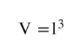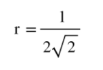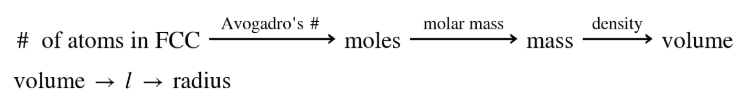# Problem: Rhodium has density of 12.43 g/cm3 and crystallizes with the face-centered cubic unit cell.Calculate the radius of a rhodium atom.

###### FREE Expert Solution
• We will be needing the info about how many atoms are there in an FCC crystal, Avogadro's number, molar mass of rhodium and the given density (12.43 g/cm3) . This will give us the volume per unit cell, eventually the radius of the cell
• From this volume, radius of an FCC unit cell can be solved by finding the l and then using the formula below• Dimensional analysis will appear as:86% (254 ratings)###### Problem Details

Rhodium has density of 12.43 g/cm3 and crystallizes with the face-centered cubic unit cell.

Calculate the radius of a rhodium atom.

Frequently Asked Questions

What scientific concept do you need to know in order to solve this problem?

Our tutors have indicated that to solve this problem you will need to apply the Unit Cell concept. You can view video lessons to learn Unit Cell. Or if you need more Unit Cell practice, you can also practice Unit Cell practice problems.

What professor is this problem relevant for?

Based on our data, we think this problem is relevant for Professor Lapeyrouse's class at UCF.